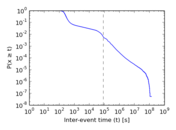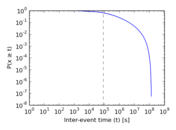# Last.fm songs

This bipartite network represent the user–song listening habits of last.fm users. Left nodes are users and right nodes are songs. An edge connects users and songs they have listened to.

 Code `Ls` Internal name `lastfm_song` Name Last.fm songs Data source http://www.dtic.upf.edu/~ocelma/MusicRecommendationDataset/lastfm-1K.html AvailabilityDataset is available for download Consistency checkDataset passed all tests Category Interaction network Node meaning User, song Edge meaning Listening Network formatBipartite, undirected Edge typeUnweighted, multiple edges Temporal dataEdges are annotated with timestamps

## Statistics

 Size n = 1,085,612 Left size n1 = 992 Right size n2 = 1,084,620 Volume m = 19,150,868 Unique edge count m̿ = 4,413,834 Wedge count s = 22,053,978,162 Claw count z = 139,516,276,582,416 Cross count x = 1,074,730,261,041,871,232 Square count q = 32,357,367,219 4-Tour count T4 = 347,084,940,232 Maximum degree dmax = 183,103 Maximum left degree d1max = 183,103 Maximum right degree d2max = 17,601 Average degree d = 35.281 2 Average left degree d1 = 19,305.3 Average right degree d2 = 17.656 8 Fill p = 0.004 102 29 Average edge multiplicity m̃ = 4.338 83 Size of LCC N = 1,085,611 Diameter δ = 6 50-Percentile effective diameter δ0.5 = 3.487 12 90-Percentile effective diameter δ0.9 = 3.897 45 Median distance δM = 4 Mean distance δm = 3.952 16 Gini coefficient G = 0.908 531 Balanced inequality ratio P = 0.111 067 Left balanced inequality ratio P1 = 0.289 593 Right balanced inequality ratio P2 = 0.161 995 Relative edge distribution entropy Her = 0.737 931 Power law exponent γ = 2.713 84 Tail power law exponent γt = 1.921 00 Degree assortativity ρ = −0.114 597 Degree assortativity p-value pρ = 0.000 00 Spectral norm α = 7,516.29 Algebraic connectivity a = 0.518 714 Spectral separation |λ1[A] / λ2[A]| = 1.043 10 Controllability C = 1,083,628 Relative controllability Cr = 0.998 172

## Plots

### Fruchterman–Reingold graph drawing### Degree distribution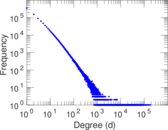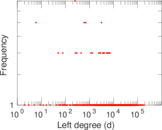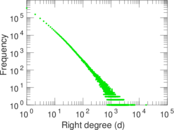### Cumulative degree distribution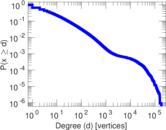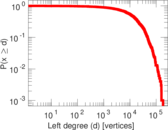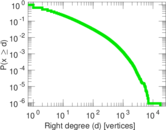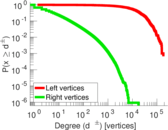### Lorenz curve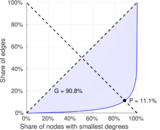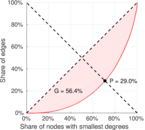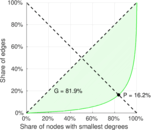### Spectral distribution of the adjacency matrix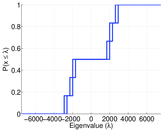### Spectral distribution of the normalized adjacency matrix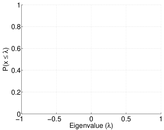### Spectral distribution of the Laplacian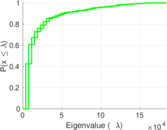### Spectral graph drawing based on the adjacency matrix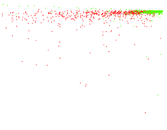### Spectral graph drawing based on the Laplacian### Spectral graph drawing based on the normalized adjacency matrix### Degree assortativity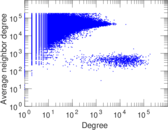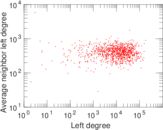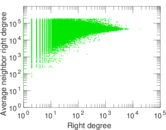### Zipf plot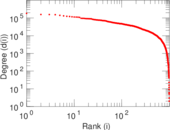### Hop distribution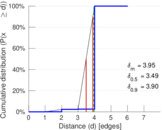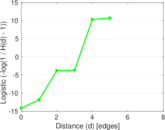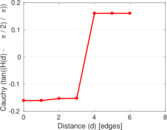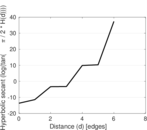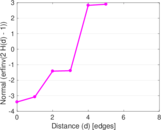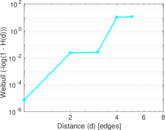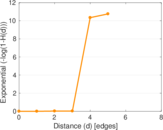### Edge weight/multiplicity distribution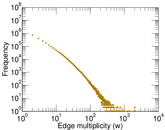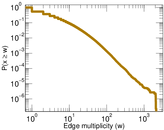### Temporal distribution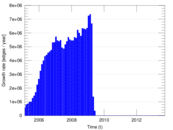### Diameter/density evolution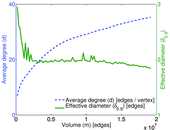### Inter-event distribution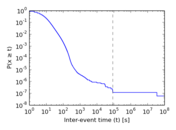### Node-level inter-event distribution